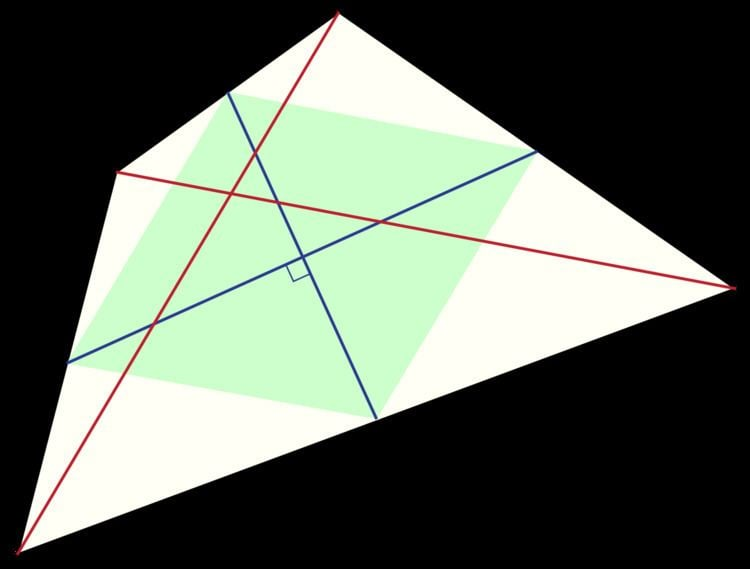# Equidiagonal quadrilateral

Updated on
Covid-19In Euclidean geometry, an equidiagonal quadrilateral is a convex quadrilateral whose two diagonals have equal length. Equidiagonal quadrilaterals were important in ancient Indian mathematics, where quadrilaterals were classified first according to whether they were equidiagonal and then into more specialized types.

## Special cases

Examples of equidiagonal quadrilaterals include the isosceles trapezoids, rectangles and squares.

Among all quadrilaterals, the shape that has the greatest ratio of its perimeter to its diameter is an equidiagonal kite with angles π/3, 5π/12, 5π/6, and 5π/12.

## Characterizations

A convex quadrilateral is equidiagonal if and only if its Varignon parallelogram, the parallelogram formed by the midpoints of its sides, is a rhombus. An equivalent condition is that the bimedians of the quadrilateral (the diagonals of the Varignon parallelogram) are perpendicular.

A convex quadrilateral with diagonal lengths p and q and bimedian lengths m and n is equidiagonal if and only if

p q = m 2 + n 2 .

## Area

The area K of an equidiagonal quadrilateral can easily be calculated if the length of the bimedians m and n are known. A quadrilateral is equidiagonal if and only if

K = m n .

This is a direct consequence of the fact that the area of a convex quadrilateral is twice the area of its Varignon parallelogram and that the diagonals in this parallelogram are the bimedians of the quadrilateral. Using the formulas for the lengths of the bimedians, the area can also be expressed in terms of the sides a, b, c, d of the equidiagonal quadrilateral and the distance x between the midpoints of the diagonals as

K = 1 4 ( 2 ( a 2 + c 2 ) 4 x 2 ) ( 2 ( b 2 + d 2 ) 4 x 2 ) .

Other area formulas may be obtained from setting p = q in the formulas for the area of a convex quadrilateral.

## Relation to other types of quadrilaterals

A parallelogram is equidiagonal if and only if it is a rectangle, and a trapezoid is equidiagonal if and only if it is an isosceles trapezoid. The cyclic equidiagonal quadrilaterals are exactly the isosceles trapezoids.

There is a duality between equidiagonal quadrilaterals and orthodiagonal quadrilaterals: a quadrilateral is equidiagonal if and only if its Varignon parallelogram is orthodiagonal (a rhombus), and the quadrilateral is orthodiagonal if and only if its Varignon parallelogram is equidiagonal (a rectangle). Equivalently, a quadrilateral has equal diagonals if and only if it has perpendicular bimedians, and it has perpendicular diagonals if and only if it has equal bimedians. Silvester (2006) gives further connections between equidiagonal and orthodiagonal quadrilaterals, via a generalization of van Aubel's theorem.

Quadrilaterals that are both orthodiagonal and equidiagonal, and in which the diagonals are at least as long as all of the quadrilateral's sides, have the maximum area for their diameter among all quadrilaterals, solving the n = 4 case of the biggest little polygon problem. The square is one such quadrilateral, but there are infinitely many others. Equidiagonal, orthodiagonal quadrilaterals have been referred to as midsquare quadrilaterals because they are the only ones for which the Varignon parallelogram (with vertices at the midpoints of the quadrilateral's sides) is a square. Such a quadrilateral, with successive sides a, b, c, d, has area

K = a 2 + c 2 + 4 ( a 2 c 2 + b 2 d 2 ) ( a 2 + c 2 ) 2 4 .

## References

Topics

 B i Link H2
 L